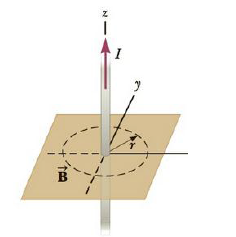Chapter 19, Problem 44P

Chapter
Section
Textbook Problem

A long, straight wire going through the origin is carrying a current of 3.00 A in the positive z-direction (Fig. P19.44). At a point a distance r = 1.20 m from the origin on the positive x-axis, find the (a) magnitude and (b) direction of the magnetic field. At a point the same distance from the origin on the negative y-axis, find the (c) magnitude and (d) direction of the magnetic field.Figure P19.44

a)

To determine
The magnitude of the magnetic field.

Explanation

Given info: The current in the long, straight wire going through the origin is 3.00A . The direction of the current is in positive z-direction. The point where the measurement has to be done is at 1.20m from the origin on the positive x-axis.

Explanation:

The magnetic field due to the long straight conductor will be,

B=μ0I2πr

• μ0 is the permeability of free space
• I is the current
• r is the distance of the point from the straight conductor

Substitute 4π×107TmA-1 for μ0 , 3.00A for I , 1

b)

To determine
The direction of the magnetic field.

c)

To determine
The magnitude of the magnetic field.

d)

To determine
The direction of the magnetic field.

Still sussing out bartleby?

Check out a sample textbook solution.

See a sample solution

The Solution to Your Study Problems

Bartleby provides explanations to thousands of textbook problems written by our experts, many with advanced degrees!

Get Started

Find more solutions based on key concepts